# High-pass filtering

High-pass (HP) filtering is almost universally done in fMRI studies. fMRI data has a lot of noise at low temporal frequencies (slow drift). This is also a main source of autocorrelation in fMRI time series data. HP filtering models ("captures") and removes low-frequency signals. This can be done in the time domain and in the frequency domain.
Filtering can also be done at the same time as fitting a linear model, by adding nuisance covariates to the design matrix, or it can be done before analysis by pre-filtering. Many software packages pre-filter because it's convenient, particularly for connectivity analyses where there is no experimental design matrix. Here, we'll look at a simple example of filtering by adding covariates to the design matrix.
Filtering is a linear operation, so it is possible to construct a matrix S that, when multiplied by the data (e.g., S*y), returns a filtered time series. Filtering can be used to condition time series data in multiple ways:
• High-pass filters remove low-frequency (slow) noise and pass high-freqency signals.
• Low-pass filters remove high-frequency noise and thus smooth the data.
• Band-pass filters allow only certain frequencies and filter everything else out
• Notch filters remove certain frequencies
There are also different ways of constructing filters:
• Discrete cosine transform (DCT) filters use a bank of cosine waves (we will look at this here)
• Chebyshev and Butterworth filters are advanced filters designed to optimize certain properties (e.g., prevent "ringing")
Here, we'll go over some of the basics of how high-pass filtering works.

#### Refresher: Sine waves

The sine wave is an oscillation, which can be described mathematically as: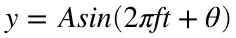...where fis the frequency or the speed of the oscillation described in the number of cycles per unit time (e.g., second), tis the the time, and θis the phase in radians. y is the signal value, and it can be a vector. If time is in units of seconds, frequency in units of 1/time, or hertz (𝐻𝑧). Amplitude 𝐴 determines the height of the waves: 𝐴 is half the distance of the peak to the trough.
Here we will plot a simple sine wave. Try playing with the different parameters (i.e., amplitude, frequency, & theta) to gain an intuition of how they each impact the shape of the wave.
More detailed explanations can be found on Michael X. Cohen's excellent Youtube channel and in his book, Analying Neural Data Analysis: Theory and Practice. Here is a picture from one of Mike's videos that shows the relationship between time domain signals (top row) and frequency domain signals (bottom row) for some simple sine waves: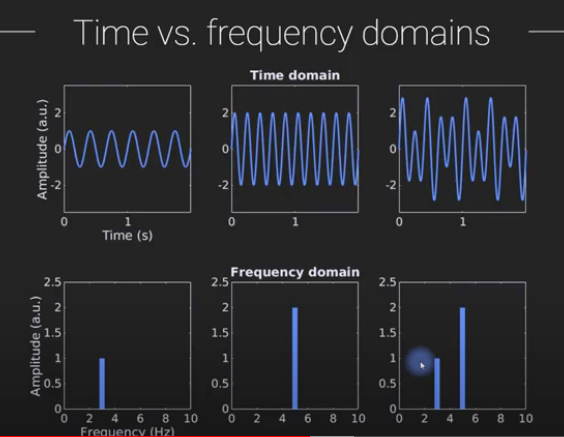First, we'll create an anonymous function in Matlab that lets us plug in any values for A, f, and θ. We'll fix the value of t to be a vector from 0 to 360 seconds, about the length of a typical fMRI run. This will be sampled at a high rate (100 Hz!), though fMRI data will be collected much slower, around 2 sec per BOLD image or 1/2 Hz for old studies or 0.5 sec per image (2 Hz) for newer multiband imaging studies. (The actual sampling rate is the Repetition Time, TR).
t = 0:.01:360; % time samples for the underlying signal
sinwave = @(A, F, theta) A .* sin(2 * pi * F * t + theta);
1. Create a signal with amplitude 2 and a 20-sec period and plot it.
Hints: Play around with the input parameters. Your signal will look strange if it's oscillating too fast to see clearly. Period is 1/F. e.g., to create a wave with amplitude 3 and 10 sec period, try this:
y = sinwave(3, .1, 0);
figure; plot(y); xlabel('Time'); ylabel('Signal');
2. What happens when you shift the phase over by pi/2 radians? Plot it. What kind of wave looks just like this? (e.g., not a sine wave, but a...)
3. Create a signal that sums three sine waves, with 1.5 sec, 20 sec, and 50 sec periods, and amplitudes of 1, 2, and 3, respectively.
Hint: It should look like this: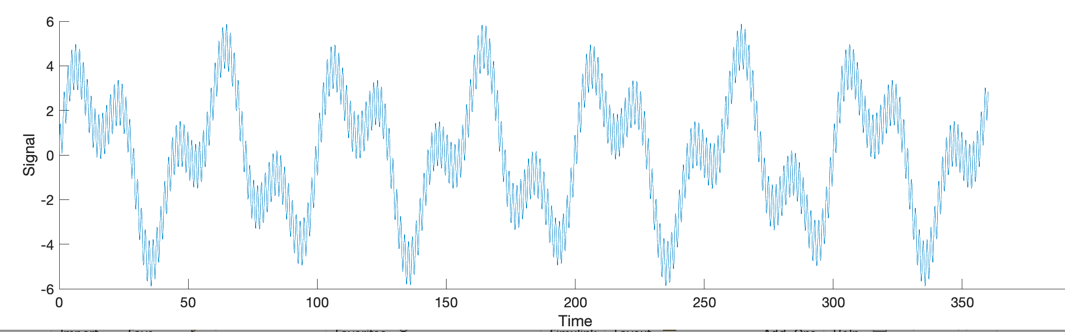And you can sum sin waves like this:
y1 = sinwave(1, 1/5, 0); % F = 1/5 Hz = .2 Hz = 1/.2 sec = 5 sec period
y2 = sinwave(2, 1/30, 0); % F = 1/30 Hz = 30 sec period
y = y1 + y2;

#### Fourier Transform

Now we'll take the Fourier Transform, decomposing the signal into sin waves. The real part of this is the signal magnitude, or the power spectrum -- power across a spectrum of frequencies. The Nyquist frequency is 1/2 the sampling rate, so we'll show that too:
yf = fft(y); % Fourier transform
mag = abs(yf); % Signal magnitude (the real part)
F = 100; % Our original sampling frequency in Hz
f = F .* (0:(length(y)-1))/length(y); % define frequencies for each element of yf
nyquist = F ./ 2; % Any signal faster than Nyquist freq will be aliased
figure; plot(f, mag, 'LineWidth', 2); hold on;
xlabel('Frequency'); ylabel('Power')
h = plot_vertical_line(nyquist);
set(h, 'LineStyle', ':')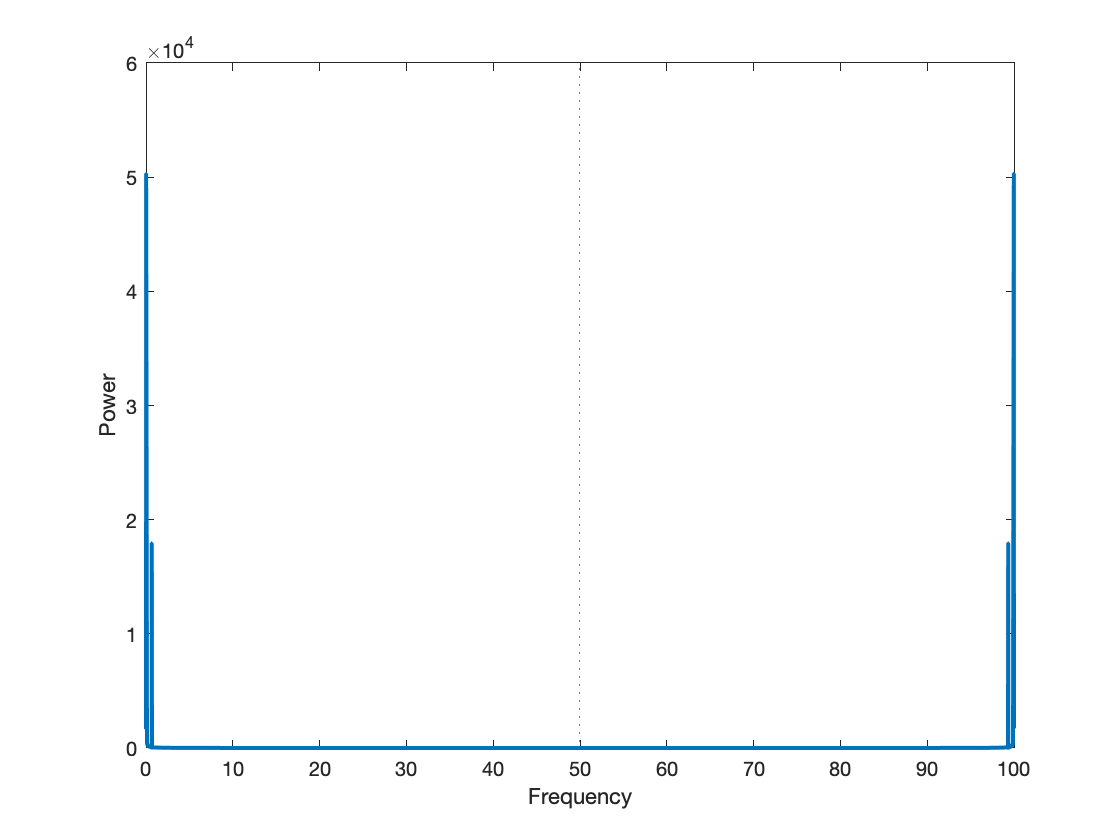Notice that the signal is reflected around the Nyquist limit. Anything moving faster than that is "invisible", as it's aliased back into the lower frequencies. Let's zoom in on the relevant part by setting the X-axis limits in the display. This is a "graphics handle" in Matlab. You can adjust figures in many ways, either through the buttons on the figure (or by clicking/scolling in the figure), or with code.
figure; plot(f, mag, 'LineWidth', 2); hold on;
xlabel('Frequency'); ylabel('Power')
set(gca, 'XLim', [0 2]) % Zoom in on frequencies from 0 (constant, fundamental frequency) to 2 Hz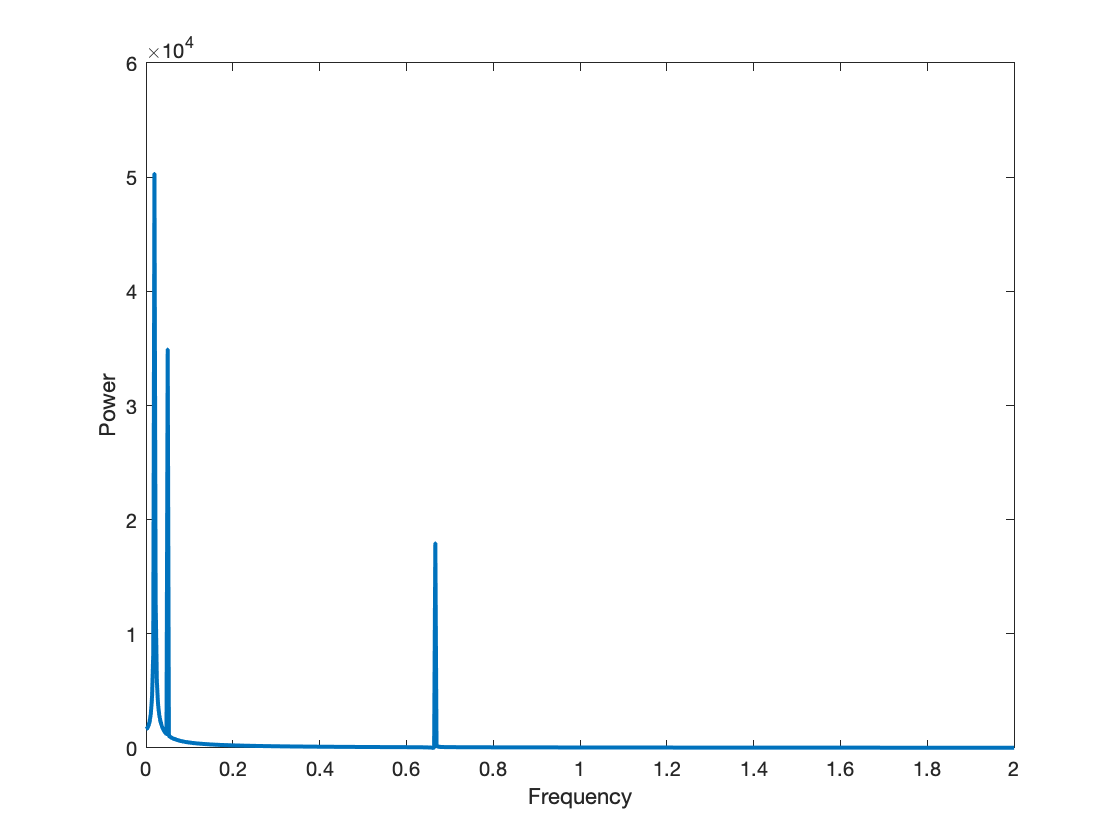It should look like this: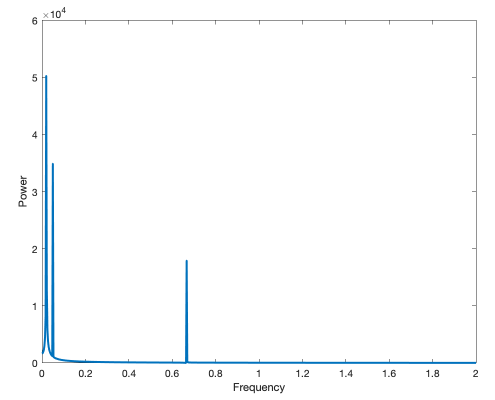#### Downsampling

In fMRI, we sample signals that have a mix of frequencies, including high-frequency oscillations, at a slower sampling rate. A simple way to downsample our signal is to take every nth sample. If our original signal is 100 Hz and we want to simulate sampling at 0.5 Hz, we'd take every 100/0.5 = 200th sample.
t_obs = t(1:200:end);
y_obs = y(1:200:end);
figure; plot(t, y); % plot underlying signal in blue
xlabel('Time'); ylabel('Signal'); hold on;
plot(t_obs, y_obs, 'LineWidth', 2, 'Color', [1 .5 0]) % plot sampled signal in orange with a heavier line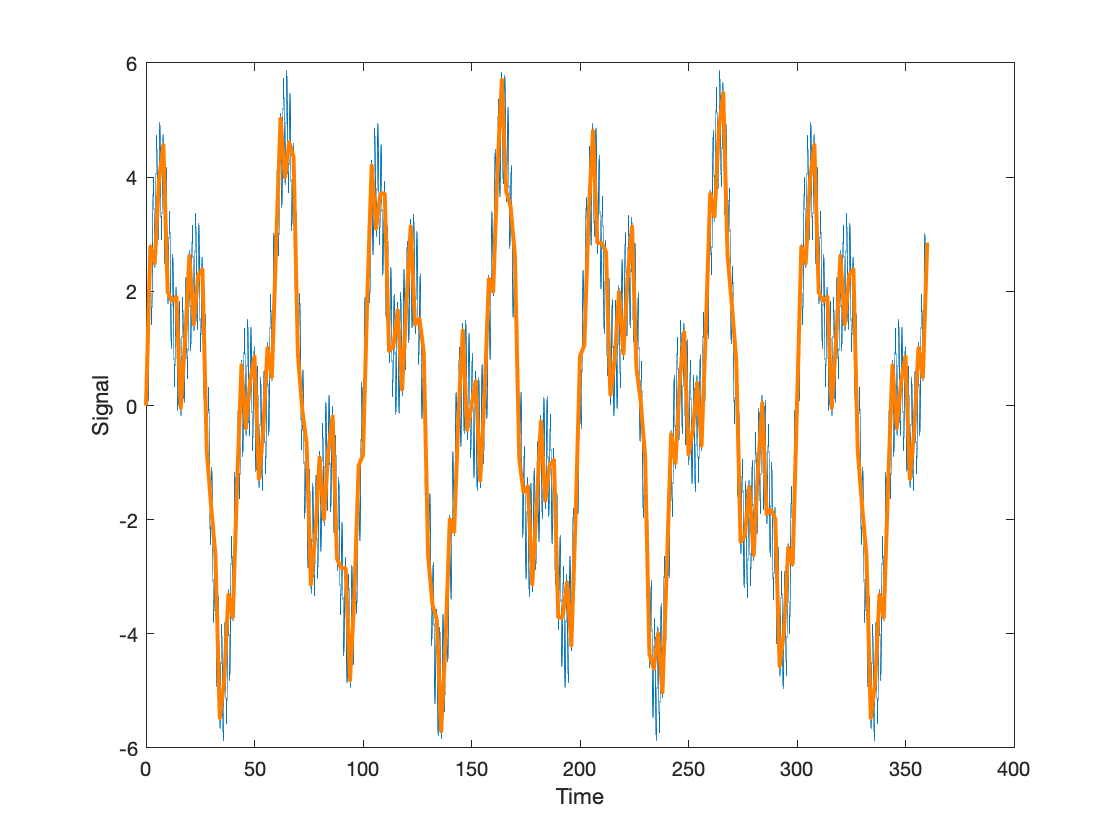1. Show the plot. What information in the signal have we lost? What is retained?
2. Do the fft on the downsampled signal, and re-create a plot of the power in the original signal, adding the new signal in orange to the plot. You can use the code below. What frequencies have been lost? Which ones have appeared? What is the Nyquist limit for the downsampled signal, and how does this help explain the plot?
figure; plot(f, mag ./ length(y), 'LineWidth', 2); hold on;
xlabel('Frequency'); ylabel('Power')
set(gca, 'XLim', [0 2])
mag_obs = abs(fft(y_obs)) ./ length(y_obs); % Signal magnitude (the real part)
f_obs = F/200 .* (0:(length(y_obs)-1))/length(y_obs); % define frequencies for each element of yf
hold on; plot(f_obs, mag_obs, 'Color', [1 .5 0], 'LineWidth', 2);
title('Frequency Domain Signal')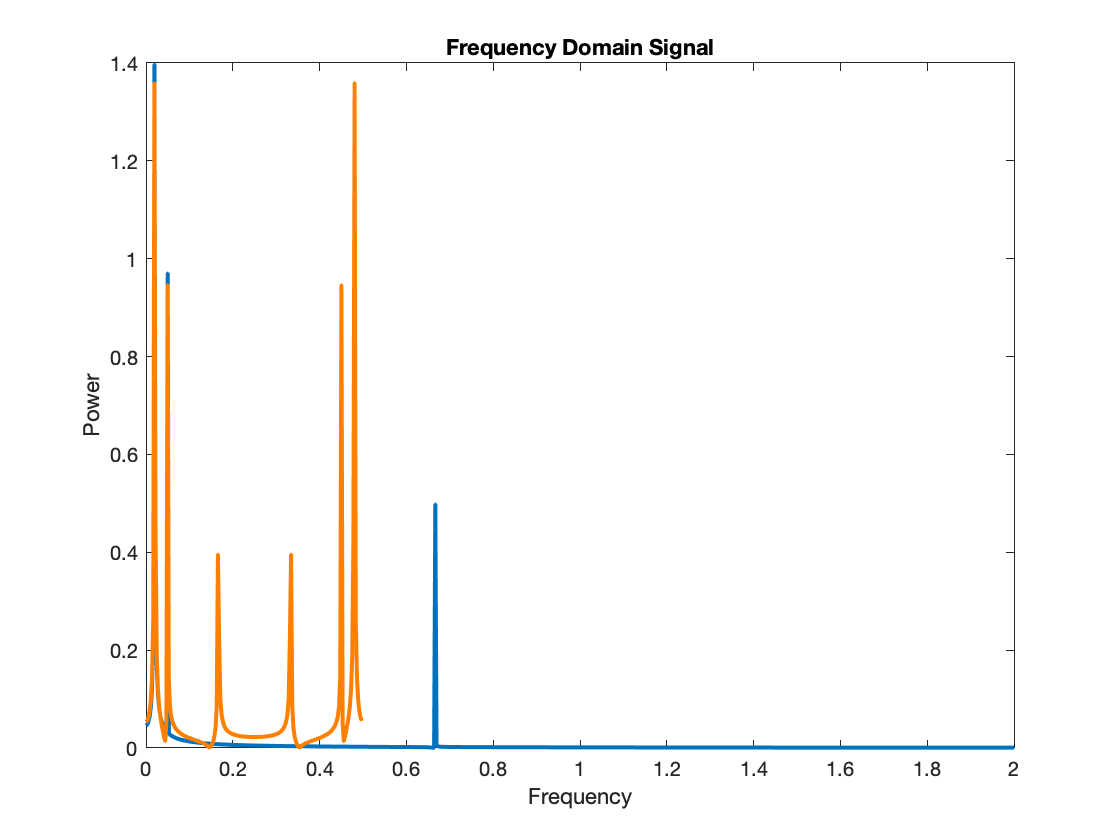### Filtering

Recall from our signal processing tutorial, that there are often other types of artifacts in fMRI signal that might take the form of slow or fast oscillations. It is common to apply a high pass filter to the data to remove low frequency artifacts. This does what it sounds like: It passes high frequencies, and removes low frequencies below a certain cutoff.
A high-pass filter has to model ("capture") and remove low-frequency signals. We can think of this in two ways: in the time domain, and in the frequency domain.
In the time domain, we'll add regressors that capture slowly-varying signals and remove their fitted responses from the data, or "residualize" the data. These could be any slowly varying curve: polynomials, splines, or others. They could also be a set of sine or cosine waves. These regressors will be added to our model (design matrix, X) as nuisance covariates. Recall the linear model equation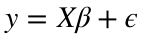. X contains regressors of interest. I can add new columns of X to capture the nuisance covariates.
In the frequency domain, we can multiply the power spectrum by a filter, which is a series of values. Where the filter values are zero, those frequencies are filtered out. These two ways of thinking about filters are different ways of representing the same process, just as the time-domain and frequency domain signals are actually just different expressions of the same signal.

#### Create an fMRI design and add high-pass filter covariates

Let's create a simple fMRI design and use it to simulate a signal. We'll add noise with some slow drift at specific frequencies so we can unpack what's going on:
TR = 2;
create_figure('Simple fMRI study with 2-condition block design');
X = create_block_design(360, TR, 2, 18, Inf, 0);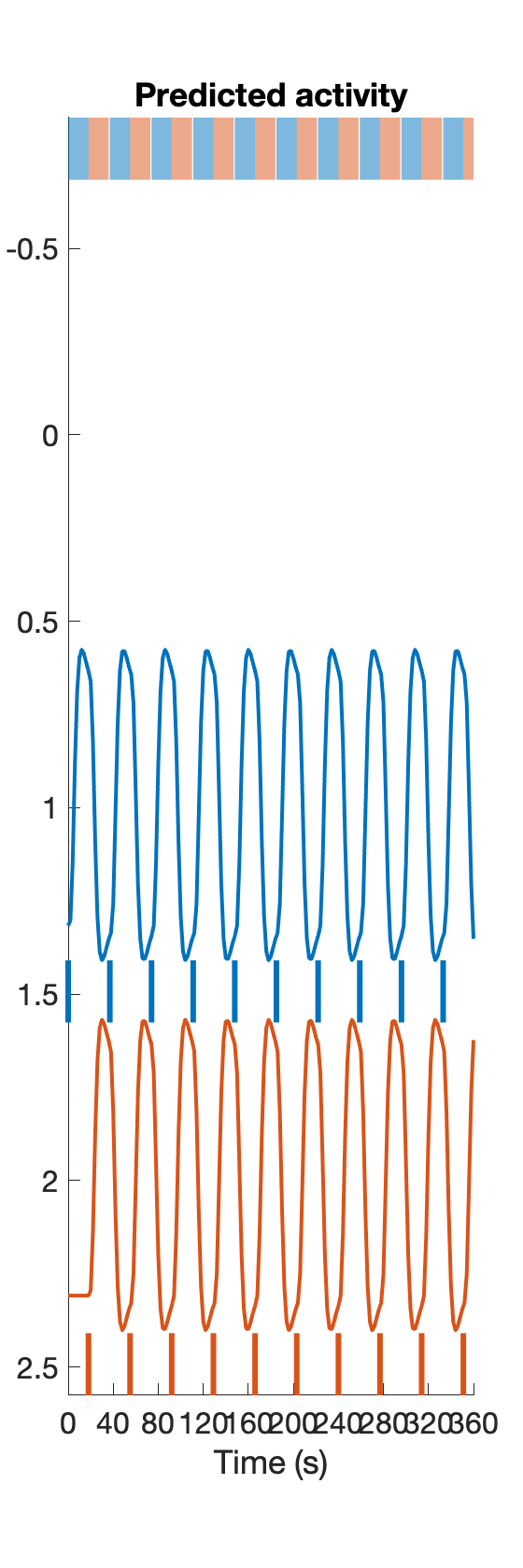% The second predictor is a mirror-image of the first, so let's save the first only as our predictor of interest
X = X(:, 1);
create_figure('2-condition block design', 3, 1);
t = 0:2:(360-1);
plot(t, X); xlabel('Time (sec)')
title('True signal: Time domain')
% Now create a signal with some faster noise and slow drift:
%y1 = sinwave(1, 1, 0); % F = 1 Hz = 1 sec. This is like a heart-beat signal. It will be aliased!
y2 = sinwave(2, 1/10, 0); % F = 1/30 Hz, 30 sec period
y3 = sinwave(5, 1/60, 0); % F = 1/30 Hz, 60 sec period
y4 = sinwave(8, 1/120, 0); % F = 1/120 Hz
y_drift = y2 + y3 + y4;
y_drift = y_drift(1:100 * TR:end - (100 * TR))'; % downsample and make a column vector
y = X + y_drift;
subplot(3, 1, 2)
plot(t, y, 'Color', 'k', 'LineWidth', 2);
title('Signal with slow drift')
% Now let's look at the FFT. We'll use periodogram( ) to simplify
[mag, f] = periodogram(X, [], [], 1/TR);
max_freq = f(mag == max(mag));
fprintf('True signal: Max power at %3.2f Hz or %3.1f sec periodicity\n', max_freq, 1/max_freq);
True signal: Max power at 0.03 Hz or 36.6 sec periodicity
subplot(3, 1, 3)
plot(f, mag, 'LineWidth', 2, 'Color', 'b');
xlabel('Frequency')
title('Frequency domain')
% Add the power spectrum for the noise:
[mag, f] = periodogram(y_drift, [], [], 1/TR);
plot(f, mag, 'LineWidth', 1, 'Color', 'k');
legend({'True signal' 'Signal+Drift'});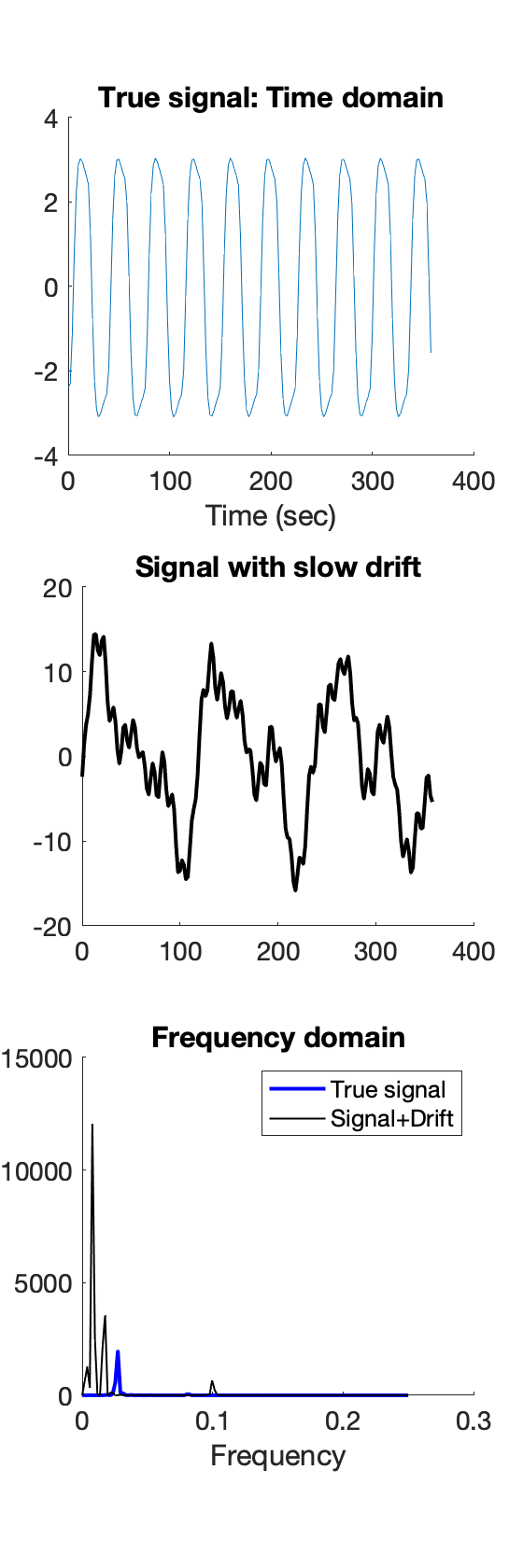Now we're ready to construct a filter matrix. discrete cosine transform (DCT), which is a basis set of cosine regressors of varying frequencies up to a filter cutoff of a specified number of seconds. Many software use 100s or 128s as a default cutoff, but we encourage caution that the filter cutoff isn’t too short for your specific experimental design. Longer trials will require longer filter cutoffs. See this paper for a more technical treatment of using the DCT as a high pass filter in fMRI data analysis. In addition, here is a more detailed discussion about filtering.
We'll use a DCT built into SPM software for this, and specifically spm_filter( ). This takes a struct variable with specific fields as input, which tells it how to construct the matrix. HParam is the filter period in seconds. TR is the samping time, as above.
K_input = struct('RT', TR, 'HParam', 100, 'row', ones(1, length(y)));
K = spm_filter(K_input);
K = K.X0;
create_figure('Filter matrix', 1, 2);
imagesc(K);
set(gca, 'YDir', 'Reverse'); axis tight
title('Heatmap view')
subplot(1, 2, 2);
plot_matrix_cols(K)
ans =
1×7 Line array: Line Line Line Line Line Line Line
xlabel('Time')
title('Plot of regressors')